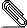# Re: Recursive Representation

```Hello Richard,

```
here is a sample attached. The ci(x) and the fj(x) should have the i resp. j as subcsripts, also inside the integral in the table. Neither the menu item nor the math symbols help...
```
Jens

Am .08.2013, 15:29 Uhr, schrieb Richard Heck <rgh...@lyx.org>:

```
```On 08/19/2013 03:07 AM, Jens-D. Doll wrote:
```
```
Hello all,

```
having formatted my document, there ist still a feature I'm missing, whci could be named multi subscription. It means the usage of subscripted characters in a text, which is already subscripted. Neither the menu item nor the math box helped for this purpose up to now.
```
```
Can somebody tell me how to format sum(bk(x)), where the sum is the greek symbol and k should be a subsript.
```
```
I'm not sure I understand you correctly. But it is easy to put subscripts on subscripts. I always type "_" (the LaTeX equivalent) to get subscripts. So you can type: b_k_x, and you will have something of this sort.
```
```
If this is not what you want, you might post a LyX file that is as close as you can get, and then explain what isn't right.
```
Richard

```Sample.lyx
Description: Binary data